Courses

# RD Sharma Solutions - Ex-6.1, Exponents, Class 7, Math Class 7 Notes | EduRev

## Class 7: RD Sharma Solutions - Ex-6.1, Exponents, Class 7, Math Class 7 Notes | EduRev

The document RD Sharma Solutions - Ex-6.1, Exponents, Class 7, Math Class 7 Notes | EduRev is a part of the Class 7 Course RD Sharma Solutions for Class 7 Mathematics.
All you need of Class 7 at this link: Class 7

Q1. Find the values of each of the following :

(i) 132

(ii) 73

(iii) 34

Sol:

(i) 132 = 13×13

= 169

(ii) 73 = 7×7×7

= 4

(iii) 34 = 3×3×3×3

= 81

Q2. Find the value of each of the following :

(i) (−7)2

(ii) (−3)4

(iii) (−5)5

Sol:

We know that if ‘a’ is a natural number, then

(−a) even number = positive number

(−a) odd number = negative number

We have,

(i) (−7)2 = (-7) ×(-7)

= 49

(ii) (−3)4 = (-3) ×(-3) ×(-3) ×(-3)

= 81

(iii) (−5)5 = (-5) ×(-5) ×(-5) ×(-5) ×(-5)

= -3125

Q3. Simply :

(i) 3×102

(ii) 22×53

(iii) 33×52

Sol:

(i) 3×102 = 3×10×10

= 3×100

= 300

(ii) 22×53 = 2×2×5×5×5

= 4×125

= 500

(iii) 33×52 = 3×3×3×5×5

= 27×25

= 675

Q4. Simply :

(i) 32×104

(ii) 24×32

(iii) 52×34

Sol:

(i) 32×104 = 3×3×10×10×10×10

= 9×10000

= 90000

(ii) 24×32 = 2×2×2×2×3×3

= 16×9

= 144

(iii) 52×34 = 5×5×3×3×3×3

= 25×81

= 2025

Q5. Simply :

(i) (−2)×(−3)3

(ii) (−3)2×(−5)3

(iii) (−2)5×(−10)2

Sol:

(i) (−2)×(−3)3 = (-2) ×(-3) ×(-3) ×(-3)

= (-2) ×(-27)

= 54

(ii) (−3)2×(−5)3 = (-3) ×(-3) ×(-5) ×(-5) ×(-5)

= 9×(-125)

= -1125

(iii) (−2)5×(−10)2 = (-2) ×(-2) ×(-2) ×(-2) ×(-2) ×(-10) ×(-10)

= (-32) × 100

= -3200

Q6. Simply :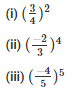Sol: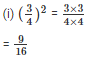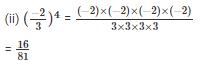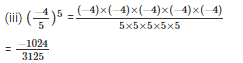Q7. Identify the greater number in each of the following

(i) 25 or 52

(ii) 34 or 43

(iii) 35 or 53

Sol:

(i) 25 or 52

= 25 = 2×2×2×2×2

= 32

= 52 = 5×5

= 25

Therefore, 25 52

(ii) 34 or 43

= 34 = 3×3×3×3

= 81

= 43 = 4×4×4

= 64

Therefore, 34 43

(iii) 35 or 53

= 35 = 3×3×3×3×3

= 243

= 53 = 5×5×5

= 125

Therefore, 35 53

(iii) 35 or 53

= 35 = 3×3×3×3×3

= 243

= 53 = 5×5×5

= 125

Therefore, 35 53

Q8. Express each of the following in exponential form

(i) (-5) ×(-5) ×(-5)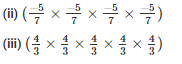Sol: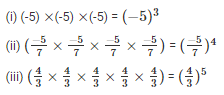Q9. Express each of the following in exponential form

(i) x ×x ×x ×x ×a ×a ×b ×b ×b

(ii) (-2) ×(-2) ×(-2) ×(-2) ×a×a×a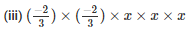Sol:

(i) x ×x ×x ×x ×a ×a ×b ×b ×b  = x4a2b3

(ii) (-2) ×(-2) ×(-2) ×(-2) ×a×a×a = (−2)4a3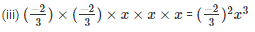Q10. Express each of the following numbers in exponential form

(i) 512

(ii) 625

(iii) 729

Sol:

(i) 512 = 29

(iii) 625 = 54

(iii) 729 = 36

Q11. Express each of the following numbers as a product of powers of their prime factors

(i) 36

(ii) 675

(iii) 392

Sol:

(i) 36 = 2×2×3×3

= 22×32

(ii) 675 = 3×3×3×5×5

= 33×52

(iii) 392 = 2×2×2×7×7

= 23×72

Q12. Express each of the following numbers as a product of powers of their prime factors

(i) 450

(ii) 2800

(iii) 24000

Sol:

(i) 450 = 2×3×3×5×5

= 2×32×52

(ii) 2800 = 2×2×2×2×5×5×7

= 24×52×7

(iii) 24000 = 2×2×2×2×2×2×3×5×5×5

= 25×3×53

Q13. Express each of the following as a rational number of the form p/q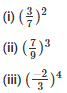Sol: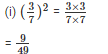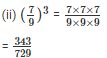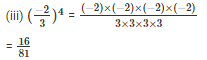Q14. Express each of the following rational numbers in power notation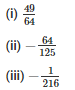Sol: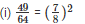Because 72 = 49 and 82 = 64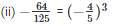Because 43 = 64 and 53 = 125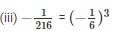Because 13 = 1 and 63 = 216

Q15. Find the value of the following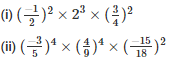Sol: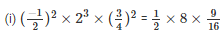= 9/8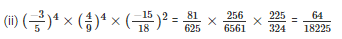Q16. If a= 2 and b = 3, the find the values of each of the followimg

(i) (a+b)a

(ii) (ab)b

(iii) (b/a)b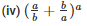Sol:

(i) (a+b)a = (2+3)2

= (5)2

= 25

(ii) (ab)b = (2×3)3

= (6)3

= 216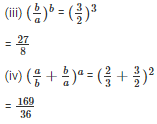The document RD Sharma Solutions - Ex-6.1, Exponents, Class 7, Math Class 7 Notes | EduRev is a part of the Class 7 Course RD Sharma Solutions for Class 7 Mathematics.
All you need of Class 7 at this link: Class 7Use Code STAYHOME200 and get INR 200 additional OFF Use Coupon Code
All Tests, Videos & Notes of Class 7: Class 7

### Top Courses for Class 7## RD Sharma Solutions for Class 7 Mathematics

97 docs

### Top Courses for Class 7Track your progress, build streaks, highlight & save important lessons and more!

,

,

,

,

,

,

,

,

,

,

,

,

,

,

,

,

,

,

,

,

,

,

,

,

,

,

,

,

,

,

;